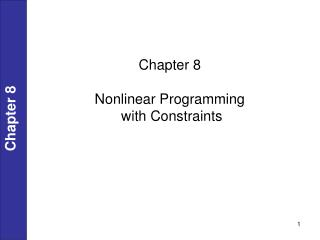DownloadDownload PresentationChapter 8

# Chapter 8

Download Presentation## Chapter 8

- - - - - - - - - - - - - - - - - - - - - - - - - - - E N D - - - - - - - - - - - - - - - - - - - - - - - - - - -
##### Presentation Transcript

1. Chapter 8 Nonlinear Programming with Constraints Chapter 8

2. Chapter 8

3. Chapter 8

4. Methods for Solving NLP Problems Chapter 8

5. ; see Fig. E 8.1a Chapter 8

6. Chapter 8

7. Chapter 8

8. Chapter 8

9. Chapter 8

10. Chapter 8

11. Chapter 8

12. Chapter 8 Note that there are n + m equations in the n + m unknowns x and λ

13. Chapter 8

14. Chapter 8

15. Chapter 8

16. Chapter 8

17. By the Lagrange multiplier method. Solution: The Lagrange function is Chapter 8 The necessary conditions for a stationary point are

18. Chapter 8

19. Chapter 8

20. Chapter 8

21. Chapter 8

22. for handling inequality constraints Chapter 8 Note g must be >0 ; r 0

23. Chapter 8 The logarithmic barrier function formulation for m constraints is

24. Chapter 8

25. Chapter 8

26. Chapter 8

27. Chapter 8

28. Use xc = 2 yc = 2 for linearization Chapter 8 (step bounds)

29. Chapter 8

30. Chapter 8

31. Chapter 8

32. Quadratic Programming (QP) Chapter 8

33. 8.3 QUADRATIC PROGRAMMING Chapter 8

34. Use of Quadratic Programming to Design Multivariable Controllers(Model Predictive Control) • Targets (set points) selected by real-time optimization software based on current operating and economic conditions • Minimize square of deviations between predicted future outputs and specific reference trajectory to new targets using QP • Framework handles multiple input, multiple output (MIMO) control problems with constraints on manipulated and controlled variables. Dynamics obtained from transfer function model. Chapter 8

35. Successive Quadratic Programming • Considered by some to be the best general nonlinear programming algorithm • Repetitively approximates nonlinear objective function with quadratic function and nonlinear constraints with linear constraints • Uses line search rather than QP step for each iteration • Inequality constrained Quadratic Programming (IQP) keeps all inequality constraints • Equality constrained Quadratic Programming (EQP) only keeps equality constraints by utilizing and active set strategy • SQP is an Infeasible Path method Chapter 8

36. Chapter 8

37. Chapter 8 solve for

38. Generalized Reduced Gradient (GRG) Chapter 8

39. Chapter 8

40. Chapter 8

41. Chapter 8

42. Chapter 8

43. Chapter 8

44. Chapter 8

45. Chapter 8

46. Chapter 8

47. Chapter 8

48. Chapter 8

49. Chapter 8